# SSC CGL (Tier - 1) Online Exam Paper - 2016 "held on 8 September 2016" Evening Shift (Quantitative Aptitude)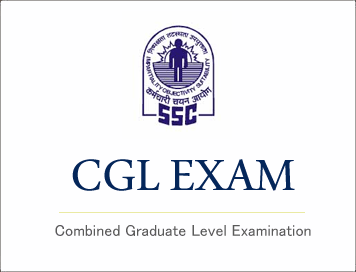## SSC CGL (Tier - 1) Online Exam Paper - 2016 "held on 8 September 2016" Evening Shift (Quantitative Aptitude)

EXAM DATE : 8-September-2016
EXAM START TIME :
16:15:00
EXAM NAME :
SSC Examination 2016

Question 51.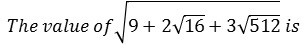Options:

1) 6
2) 5
3) 2√8
4) 3√6

Question 52.The lengths of diagonals of a rhombus are 24cm and 10cm the perimeter of the rhombus (in cm ) is :

Options:

1) 52
2) 56
3) 68
4) 72

Question 53.A dishonest shopkeeper professes to sell goods at his cost price but uses a false weight of 950 gms, for each kilogram. His gain percentage isQuestion 54.Ratio of A's age to B's age is 4:3. 'A' will be 26 years old after 6 years. The age of B now is

Options:

1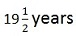2) 12 years
3) 21 years
4) 15 years
Correct Answer: 15 years

Question 55.If the Arithmetic mean of 7, 5, 13, x and 9 is 10, then the value of x is

Options:

1) 10
2) 12
3) 14
4) 16

Question 56.A man sells an article at 15% profit. If he had sold it for ₹ 6 more, he would have gained 18%. The man bought the article for

Options:

1) 100
2) 150
3) 200
4) 250

## Study Kit for SSC CGL EXAM

Question 57.A's salary was decreased by 50% and subsequently increased by 50%. How much percent does he lose?

Options:

1) 25%
2) 50%
34) No loss

Question 58.A car moving in the morning fog passes a man walking at 4km/h. in the same direction. The man can see the car for 3 minutes and visibility is upto a distance of 130m. The speed of the car is :

Options:

1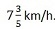23) 7km/h.
4) 5km/h.Question 59.A money lender lends Rs. 400 for 3 years to a person and lends Rs. 500 for 4 years to the other person at the same rate of simple interest. If altogether he receives Rs. 160 as interest, what is the rate of interest per annum ?

Options:

1) 5%
2) 7%
3) 9%
4) 10%

Question 60.If x = 1/(√2+1) then the value of x2 + 2x - 1 is

Options:

1) 2√2
2) 4
3) 0
4) 2

Question 61.If x + 1/x = √13, then 3x/(x2-1) equal to

Options:

1) 3√13
2) √13/3
3) 1
4) 3

Question 62.O is an centre of a circle. P is an external point of it at distance of 13cm from O. The radius of the circle is 5cm. Then the length of a tangent to the circle from P upto the point of contact is

Options:

1) √194cm
2) 10cm
3) 12cm
4) 8cm

Question 63.If θ is acute angle and sin(θ+18°)= 1/2, then the value of θ in circular measure is

Options:

Question 64.18 men or 36 boys working 6 hours a day can plough a field in 24 days. In how many days will 24 men and 24 boys working 9 hours a day plough the same field ?

Options:

1) 9
2) 10
3) 6
4) 8

Question 65.If a(x + y) = b(x - y) = 2ab, then the value of 2(x2 + y2) is

Options:

1) 2(a2 - b2)
2) 2(a2 + b2)
3) 4(a2 - b2)
4) 4(a2 + b2

Correct Answer: 4(a2 + b2)

Question 66.If x + 1/x = 6, then value of x2 + 1/x2 is

Options:

1) 23
2) 16
3) 34
4) 32

Question 67.G is the centroid of the equilateral triangle ABC, if AB = 9cm then AG is equal to

Options:

1) 3√3cm
2) 3cm
3) 3√3/2cm
4) 6cm

Question 68.In the figure (not drawn to scale) given below, if AD = DC = BC and ∠BCE = 96°,then ∠DBC is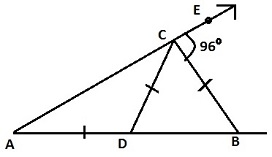Options:

1) 32°
2) 84°
3) 64°
4) 96°

Question 69.If θ is positive acute angle and 4sin2θ = 3, then the value of tan θ - cot θ/2 is

Options:

1) 1
2) 0
3) √3
4) 1/√3

Question 70.The chord AB of a circle of centre O subtends an angle θ with the tangent at A to the circle. Then measure of∠ABO is

Options:

1) θ
2) 90° - θ
3) 90° + θ
4) 2 (180° - θ)

Correct Answer: 90° - θ

Question 71.From a point on a bridge across the river, the angles of depression of the banks on opposite sides of the river are 30° and 45° respectively. If the bridge is at a height of 2.5m from the banks, then the width of the river is (take √3 = 1.732)

Options:

1) 5.83m
2) 6.83m
3) 5.76m
4) 6.87m

Question 72.The pie-chart given below show usage of materials in a construction of a house. Study the chart and answers the questions :The percentage of steel used in the construction of a house

Options:

1) 10%
2) 12%
3) 20%
4) 36%

Question 73.The pie-chart given below show usage of materials in a construction of a house. Study the chart and answers the questions :The ratio of cement and bricks used in the construction is

Options:

1) 3:4
2) 2:3
3) 4:3
4) 2:5

Question 74.The pie-chart given below show usage of materials in a construction of a house. Study the chart and answers the questions :If the cost of cement is ₹ 5000/- then the labour cost is

Options:

1) 5500
2) 6250
3) 9000
4) 4000

Question 75.The pie-chart given below show usage of materials in a construction of a house. Study the chart and answers the questions :The average of percentages of steel, cement and miscellaneous items used in the construction is

Options:

1) 10%
2) 25%
3) 20%
4) 35%